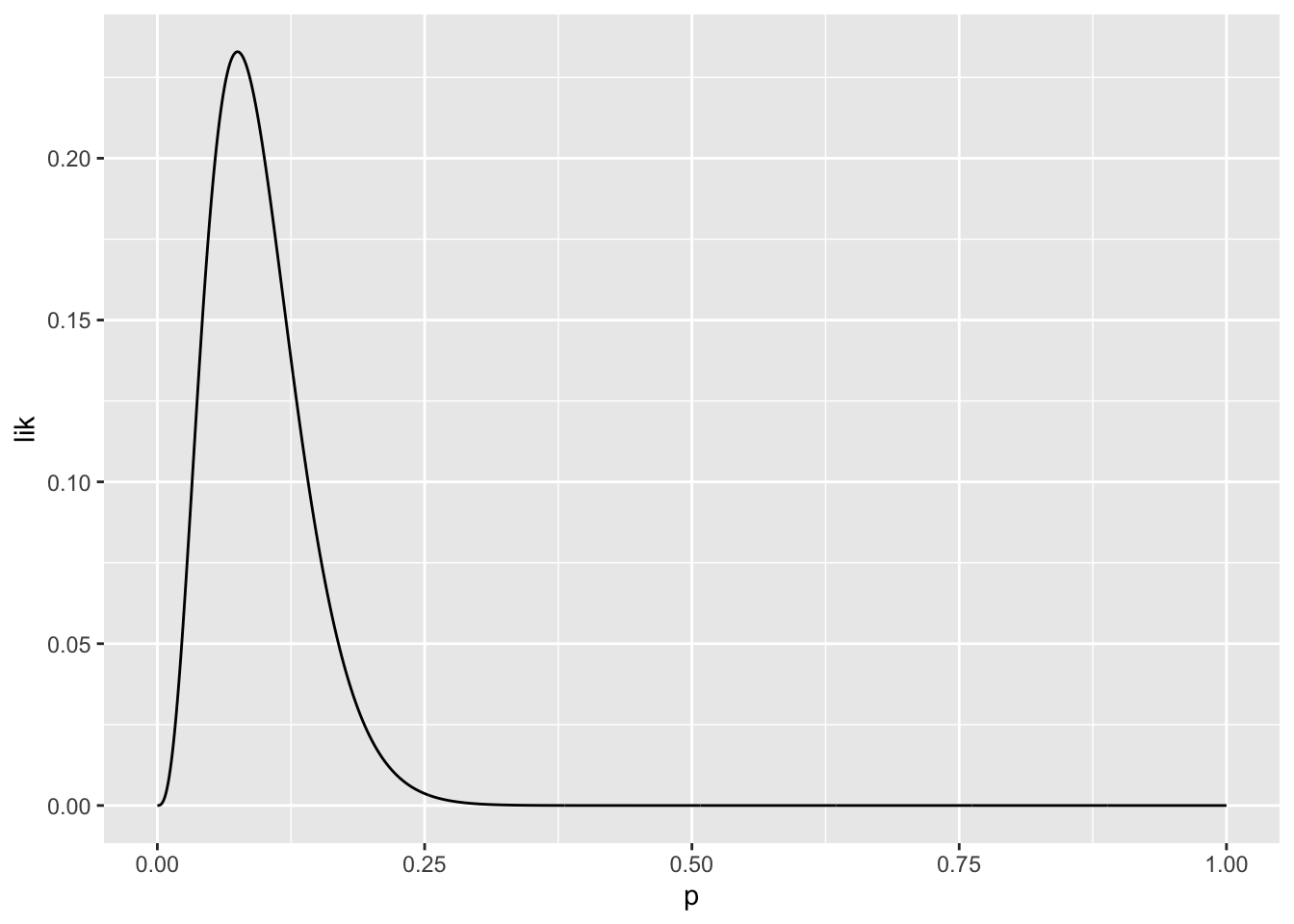Suppose that we have the following independent observations and we know that they come from the same probability density function

k<-c(39,35,34,34,24) #our observations
library('ggplot2')
dat<-data.frame(k,y=0) #we plotted our observations in the x-axis
p<-ggplot(data=dat,aes(x=k,y=y))+geom_point(size=4)
pWe don’t know the exact probability density function, but we know that the shape is the shape of the binomial distribution $f(x|\theta)=f(k|n,p)=\binom{n}{k}p^k(1-p)^{n-k}$

Let’s suppose that we know the parameter n (n=100) and we are interested in estimating p. $f(x|\theta)=f(k|p)=\binom{100}{k}p^k(1-p)^{100-k}$

Let’s plot two arbitrary probability density functions fixing the parameter p of the parametric family

kseq<-seq(20,40,1)
f1<-dbinom(kseq,100,.33) #p=.33
f2<-dbinom(kseq,100,.25) # p=.25
curve1<-data.frame(kseq,f1)
curve2<-data.frame(kseq,f2)

p<-p+geom_segment(data=curve1,aes(x=kseq,xend=kseq,y=0,yend=f1,color='p=.33'))+
geom_segment(data=curve2,aes(x=kseq+.2,xend=kseq+.2,y=0,yend=f2,color='p=.25')) #we introduce a small jitter in the distribution to avoid overplotting
pIt is clear that the probability density function $$f$$ for p=.33 describes the observations better than the other one. We are interested in the $$f$$ that maximizes $$L$$.

The joint density function is $f(k|p)=f(k_1|p)f(k_2|p)...f(k_5|p)=$ $=\binom{100}{k_1}p^{k_1}(1-p)^{100-k_1}\binom{100}{k_2}p^{k_2}(1-p)^{100-k_2}...\binom{100}{k_5}p^{k_5}(1-p)^{100-k_5}$

that when considered as a function of the parameter is $L(p|k)=L(p|39,35,34,34,24)=f(k|p)=f(39,35,34,34,24|p)=$ $=f(39|p)f(35|p)...f(24|p)=$ $=\binom{100}{39}p^{39}(1-p)^{100-39}\binom{100}{35}p^{35}(1-p)^{100-35}...\binom{100}{24}p^{24}(1-p)^{100-24}$

and $log(L)=log(f(k|p))=log(f(k_1|p))+log(f(k_2|p))+...+log(f(k_5|p))=$ $=log(f(39|n,p))+log(f(35|n,p))+...+log(f(24|n,p))$

logL<-function(p) sum(dbinom(k,100,p,log=T)) 

We can calculate the $$log(L)$$ for the two previous examples to verify that $$log(L)$$ is larger for $$\lambda=33$$

logL(.33)
##  -15.1822
logL(.25)
##  -23.59537
logL(.33)>logL(.25)
##  TRUE

Let’s plot the $$log(L)$$ as a function of p.

library('plyr')
pSeq<-seq(0,1,.001)
logLike<-laply(pSeq,function(z) logL(z))
dLogL<-data.frame(pSeq,logLike)
ggplot(data=dLogL,aes(x=pSeq,y=logLike))+geom_line()+ylim(-100,0)So it seems that values of p around .3 maximizes log(L). Let’s maximize it properly using optimize.

estPar<-optimize(logL,c(0,1),maximum=T)
MLEparameter<-estPar\$maximum
MLEparameter
##  0.3319917

So, we found that from the parametric family, the probability density function that better characterizes the observations according to MLE is the one described by the parameter p=0.3319917. Let’s plot the distribution in green in the previous graph

fMLE<-dbinom(kseq,100,MLEparameter)
curveMLE<-data.frame(kseq,fMLE)

p<-p+geom_segment(data=curveMLE,aes(x=kseq-.2,xend=kseq-.2,y=0,yend=fMLE),color='green') #we introduce a small jitter in the distribution to avoid overplotting
p